# Source Separation with Sparsity¶

Important: Please read the installation page for details about how to install the toolboxes. $\newcommand{\dotp}{\langle #1, #2 \rangle}$ $\newcommand{\enscond}{\lbrace #1, #2 \rbrace}$ $\newcommand{\pd}{ \frac{ \partial #1}{\partial #2} }$ $\newcommand{\umin}{\underset{#1}{\min}\;}$ $\newcommand{\umax}{\underset{#1}{\max}\;}$ $\newcommand{\umin}{\underset{#1}{\min}\;}$ $\newcommand{\uargmin}{\underset{#1}{argmin}\;}$ $\newcommand{\norm}{\|#1\|}$ $\newcommand{\abs}{\left|#1\right|}$ $\newcommand{\choice}{ \left\{ \begin{array}{l} #1 \end{array} \right. }$ $\newcommand{\pa}{\left(#1\right)}$ $\newcommand{\diag}{{diag}\left( #1 \right)}$ $\newcommand{\qandq}{\quad\text{and}\quad}$ $\newcommand{\qwhereq}{\quad\text{where}\quad}$ $\newcommand{\qifq}{ \quad \text{if} \quad }$ $\newcommand{\qarrq}{ \quad \Longrightarrow \quad }$ $\newcommand{\ZZ}{\mathbb{Z}}$ $\newcommand{\CC}{\mathbb{C}}$ $\newcommand{\RR}{\mathbb{R}}$ $\newcommand{\EE}{\mathbb{E}}$ $\newcommand{\Zz}{\mathcal{Z}}$ $\newcommand{\Ww}{\mathcal{W}}$ $\newcommand{\Vv}{\mathcal{V}}$ $\newcommand{\Nn}{\mathcal{N}}$ $\newcommand{\NN}{\mathcal{N}}$ $\newcommand{\Hh}{\mathcal{H}}$ $\newcommand{\Bb}{\mathcal{B}}$ $\newcommand{\Ee}{\mathcal{E}}$ $\newcommand{\Cc}{\mathcal{C}}$ $\newcommand{\Gg}{\mathcal{G}}$ $\newcommand{\Ss}{\mathcal{S}}$ $\newcommand{\Pp}{\mathcal{P}}$ $\newcommand{\Ff}{\mathcal{F}}$ $\newcommand{\Xx}{\mathcal{X}}$ $\newcommand{\Mm}{\mathcal{M}}$ $\newcommand{\Ii}{\mathcal{I}}$ $\newcommand{\Dd}{\mathcal{D}}$ $\newcommand{\Ll}{\mathcal{L}}$ $\newcommand{\Tt}{\mathcal{T}}$ $\newcommand{\si}{\sigma}$ $\newcommand{\al}{\alpha}$ $\newcommand{\la}{\lambda}$ $\newcommand{\ga}{\gamma}$ $\newcommand{\Ga}{\Gamma}$ $\newcommand{\La}{\Lambda}$ $\newcommand{\si}{\sigma}$ $\newcommand{\Si}{\Sigma}$ $\newcommand{\be}{\beta}$ $\newcommand{\de}{\delta}$ $\newcommand{\De}{\Delta}$ $\newcommand{\phi}{\varphi}$ $\newcommand{\th}{\theta}$ $\newcommand{\om}{\omega}$ $\newcommand{\Om}{\Omega}$

This numerical tour explore local Fourier analysis of sounds, and its application to source separation from stereo measurements.

In :
options(repr.plot.width=3.5, repr.plot.height=3.5)
options(warn=-1) # turns off warnings, to turn on: "options(warn=0)"

library(Matrix)
library(tuneR)
library(colorRamps)
library(audio)

# Importing the libraries
for (f in list.files(path="nt_toolbox/toolbox_general/", pattern="*.R")) {
source(paste("nt_toolbox/toolbox_general/", f, sep=""))
}
for (f in list.files(path="nt_toolbox/toolbox_signal/", pattern="*.R")) {
source(paste("nt_toolbox/toolbox_signal/", f, sep=""))
}

Attaching package: ‘audio’

The following object is masked from ‘package:tuneR’:

play

Attaching package: ‘pracma’

The following objects are masked _by_ ‘.GlobalEnv’:

The following objects are masked from ‘package:Matrix’:

expm, lu, tril, triu



## Sound Mixing¶

We load 3 sounds and simulate a stero recording by performing a linear blending of the sounds.

In :
n = 1024*16
s = 3 #number of sounds
p = 2 #number of micros

x = Matrix(0,nrow=n, ncol=3)


Normalize the energy of the signals.

In :
x = x/matrix(rep(c(sd(x[,1]),sd(x[,2]),sd(x[,3])),each=n),nrow=n)


We mix the sound using a $2\mathrm{x}3$ transformation matrix. Here the direction are well-spaced, but you can try with more complicated mixing matrices.

Compute the mixing matrix

In :
theta = seq(from=0, to =pi, by=pi/s)[-(s+1)]
theta = 0.2
M = rbind(cos(theta),sin(theta))


Compute the mixed sources.

In :
y = x %*% t(M)


Display of the sounds and their mix.

In :
options(repr.plot.width=6, repr.plot.height=3.5)
for (i in (1:s)){
plot(x[, i], main=paste("Source", i), type="l", ylab="", xlab="", col="blue")
}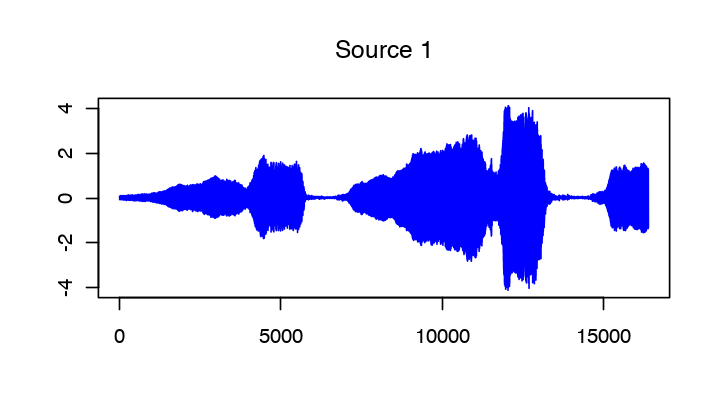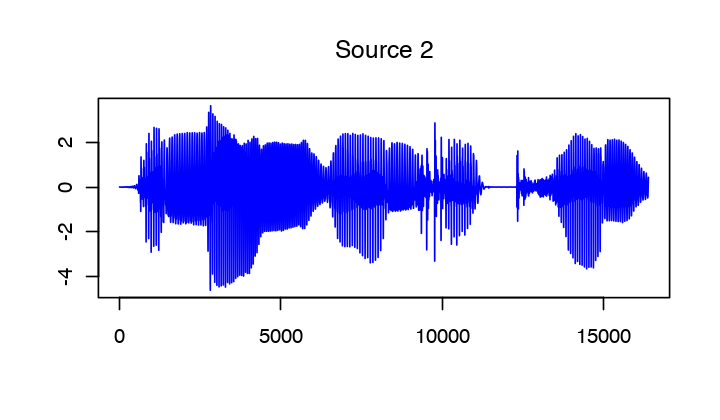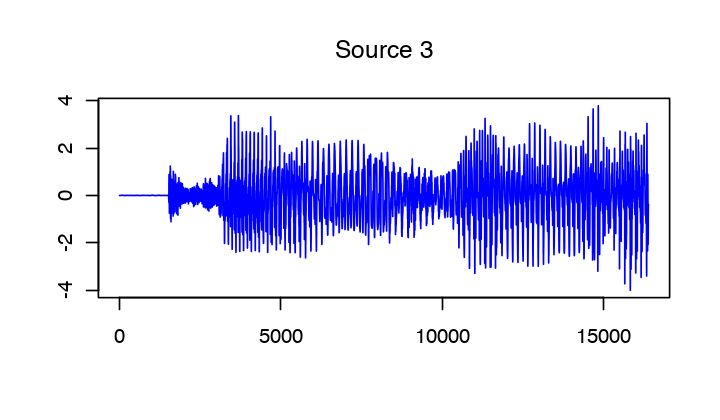Display of the micro output.

In :
options(repr.plot.width=6, repr.plot.height=3.5)
for (i in (1:p)){
plot(y[, i], main=paste("Micro", i), type="l", ylab="", xlab="", col="blue")
}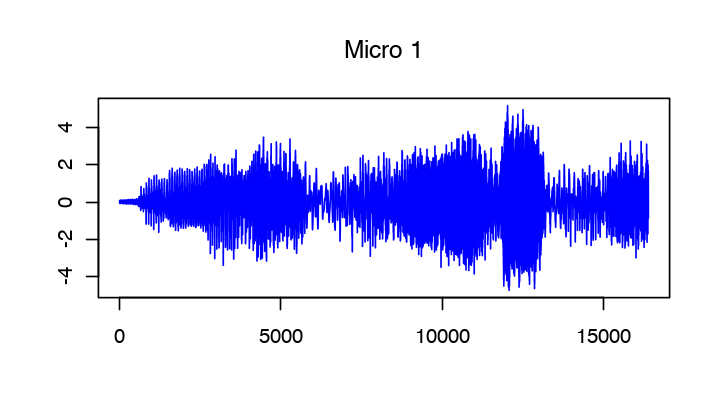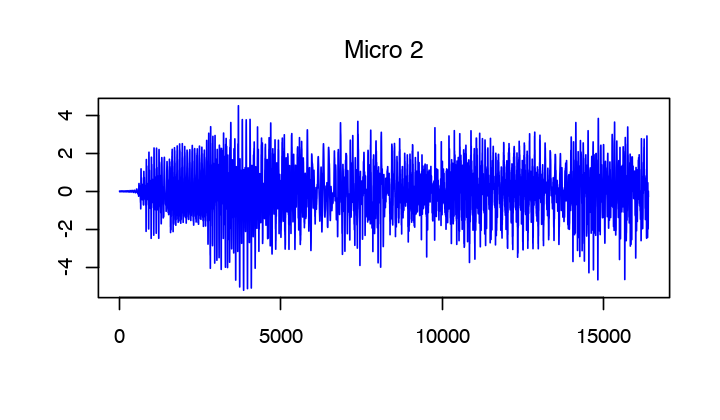## Local Fourier analysis of sound.¶

In order to perform the separation, one performs a local Fourier analysis of the sound. The hope is that the sources will be well-separated over the Fourier domain because the sources are sparse after a STFT.

First set up parameters for the STFT.

In :
w = 128   #size of the window
q = floor(w/4)  #overlap of the window


Compute the STFT of the sources.

In :
X = replicate(s, Matrix(0,nrow=w,ncol=4*w+1), simplify=FALSE)
Y = replicate(p, Matrix(0,nrow=w,ncol=4*w+1), simplify=FALSE)

for (i in (1:s))
{
X[[i]] = perform_stft(x[,i], w,q,n)
plot_spectogram(X[[i]], paste("Source", i))
}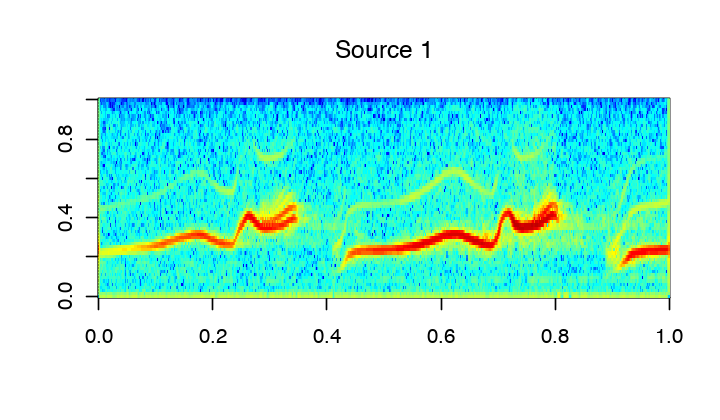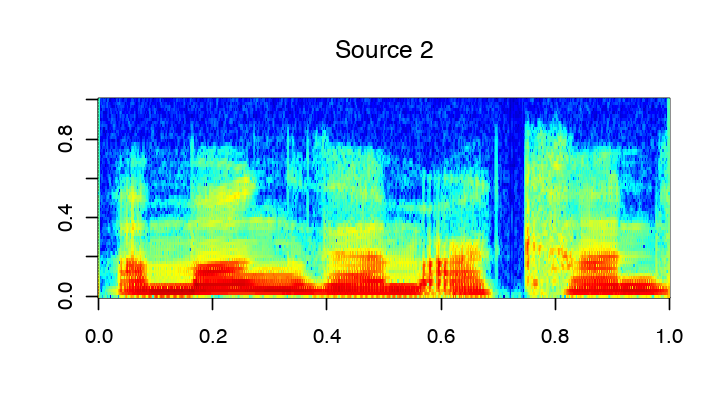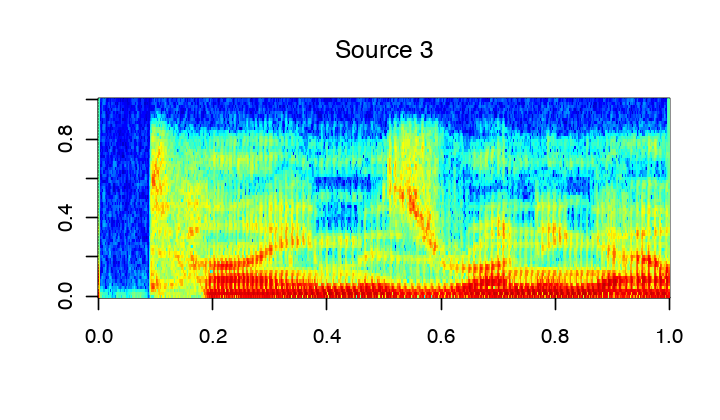Exercise 1

Compute the STFT of the micros, and store them into a matrix |Y|.

In :
source("nt_solutions/audio_separation/exo1.R")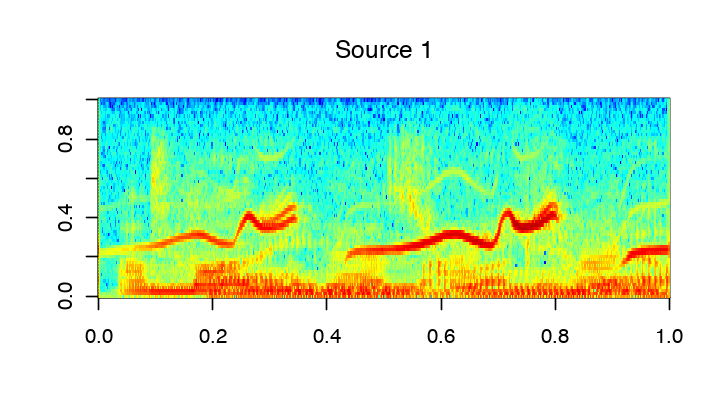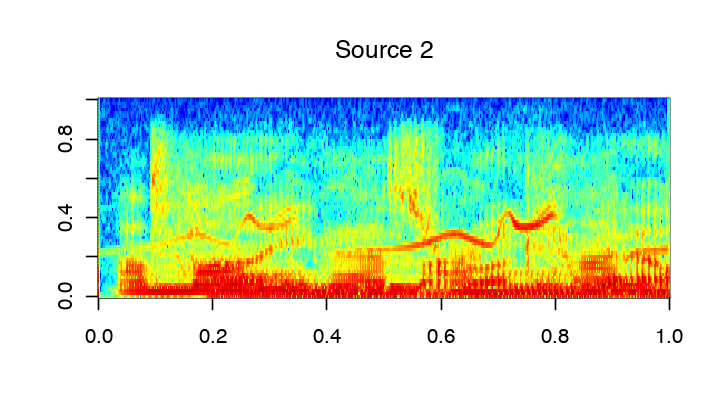In :
## Insert your code here.


## Estimation of Mixing Direction by Clustering¶

Since the sources are quite sparse over the Fourier plane, the directions are well estimated by looking as the direction emerging from a point clouds of the transformed coefficients.

First we compute the position of the point cloud.

In :
mf = dim(Y[])
mt = dim(Y[])
P = matrix(0, nrow=mt*mf, ncol=p)
c = c()
d = c()
for (j in (1:mf))
{
c = c(c,Y[][j,])
d = c(d,Y[][j,])
}
P[,1] = c
P[,2] = d
P = rbind(Re(P), Im(P))


Then we keep only the 5% points with largest energy.

Display some points in the original (spacial) domain.

Number of displayed points.

In :
npts = 6000


Display the original points.

In :
options(repr.plot.width=5, repr.plot.height=4)

sel = sample(n)

sel = sel[1:npts]

plot(y[sel,1], y[sel,2], type="p", pch=19, col = "blue", cex=0.05, main="Time domain", xlab="", ylab="")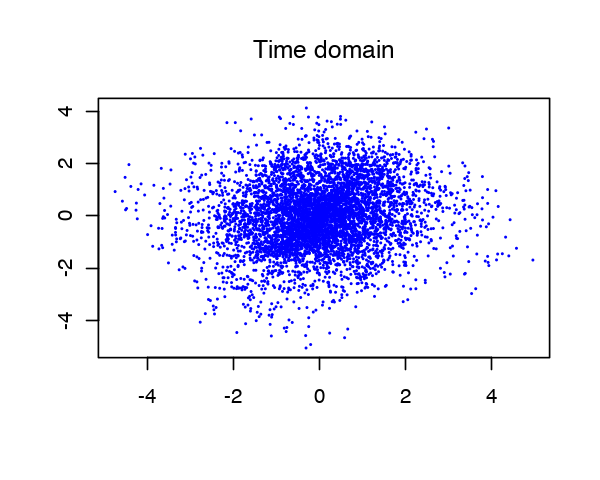Exercise 2

Display some points of $P$ in the transformed (time/frequency) domain.

In :
source("nt_solutions/audio_separation/exo2.R")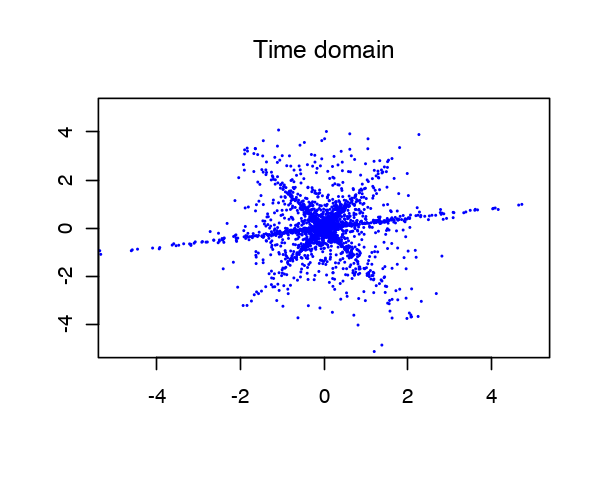In :
## Insert your code here.


We compute the angle associated to each point over the transformed domain. The histogram shows the main direction of mixing.

In :
nrow = dim(P)
Theta = c()
for (i in (1:nrow))
{
Theta[i] = atan2(P[i,2], P[i,1])%%pi
}


Display histogram.

In :
options(repr.plot.width=4, repr.plot.height=4)

nbins = 100
t = seq(from=pi/200, pi, (pi-pi/200)/(nbins-1))
hist = hist(Theta, xlim=c(min(Theta),max(Theta)), main="", breaks=nbins, plot=FALSE)
h = hist$counts/sum(hist$counts)
barplot(h, xlab="Theta", col ="DarkBlue" , tick=TRUE)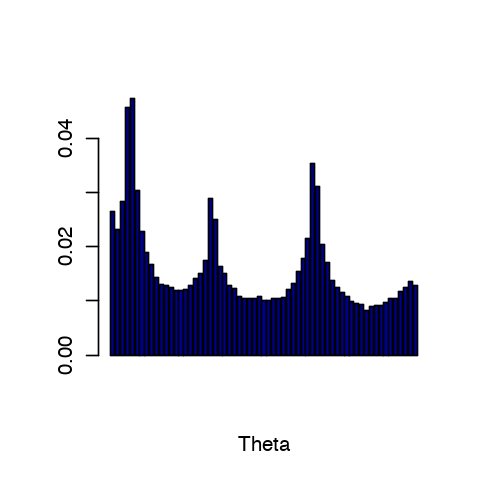Exercise 3

The histogram computed from the whole set of points are not peacked enough. To stabilize the detection of mixing direction, compute an histogram from a reduced set of point that have the largest amplitude. Compute the energy of each point. Extract only a small sub-set.

In :
source("nt_solutions/audio_separation/exo3.R")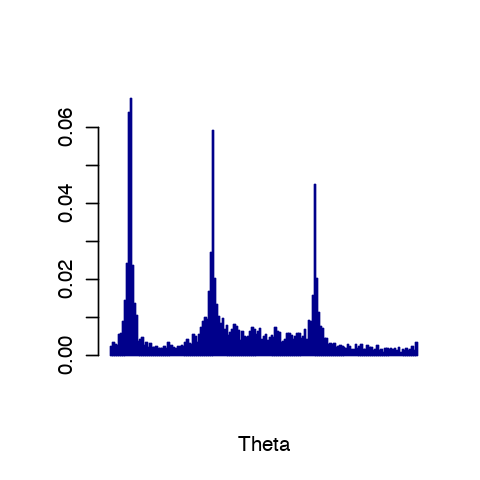In :
## Insert your code here.


Exercise 4

Detect the direction $M_1$ approximating the true direction $M$ by looking at the local maxima of the histogram. First detect the set of local maxima, and then keep only the three largest. Sort in descending order.

In :
source("nt_solutions/audio_separation/exo4.R")

          [,1]      [,2]       [,3]
[1,] 0.9800666 0.5000000 -0.5000000
[2,] 0.1986693 0.8660254  0.8660254
[,1]      [,2]       [,3]
[1,] 0.9851093 0.6730125 -0.0784591
[2,] 0.1719291 0.7396311  0.9969173

In :
## Insert your code here.


## Separation of the Sources using Clustering¶

Once the mixing direction are known, one can project the sources on the direction.

We compute the projection of the coefficients Y on each estimated direction.

In :
A = matrix(0, nrow=mt*mf, ncol=p)
c = c()
d = c()
for (j in (1:mf))
{
c = c(c,Y[][j,])
d = c(d,Y[][j,])
}
A[,1] = c
A[,2] = d


Compute the projection of the coefficients on the directions.

In :
C = abs(t(M) %*% t(A))


At each point $x$, the index $I(x)$ is the direction which creates the largest projection.

$I$ is the index of the closest source.

In :
vec = c(1:dim(C))
tmp = apply(C[,vec],2,max)
I = max.col(t(C))
I = t(matrix(I, nrow=mt, ncol=mf))


An additional denoising is achieved by removing small coefficients.

In :
T = .05
D = sqrt(abs(Y[])**2+abs(Y[])**2)
I = I*(D > T)


We can display the segmentation of the time frequency plane.

In :
options(repr.plot.width=8, repr.plot.height=2.5)
options(warn=-1) # turns off warnings, to turn on: "options(warn=0)"

image(t(I[1:floor(mf/2),]),col=matlab.like2(50),interpolation_type="nearest")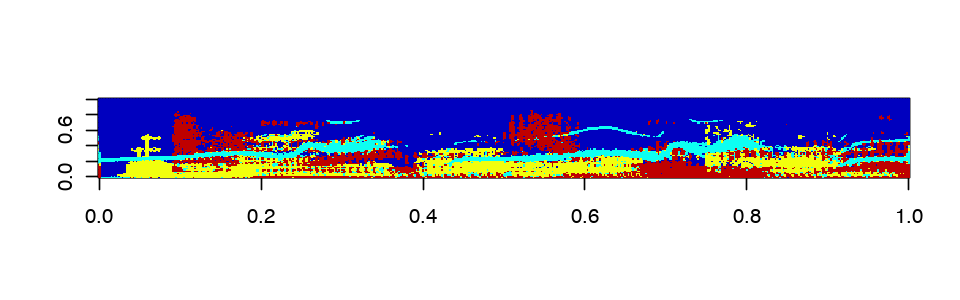The recovered coefficients are obtained by projection.

In :
Proj = t(M) %*% t(A)
Xr = replicate(s, matrix(0,nrow=w,ncol=4*w+1), simplify=FALSE)
for (i in (1:s))
{
Xr[[i]] = t(matrix(Proj[i,], nrow=mt, ncol=mf))*((I+1)==i)
}


The estimated signals are obtained by inverting the STFT.

In :
xr = Matrix(0, nrow=n, ncol=s)
for (i in (1:s))
{
xr[,i] = perform_stft(Re(Xr[[i]]), w,q,n)
}


One can display the recovered signals.

In :
options(repr.plot.width=5, repr.plot.height=3)
for (i in (1:s))
{
plot(xr[,i], main=paste("Estimated source #", i), type="l", ylab="", xlab="", col="blue")
}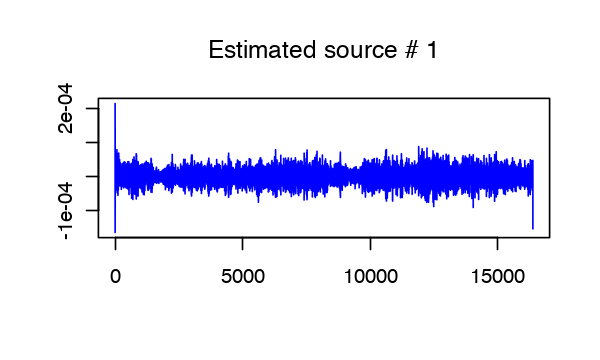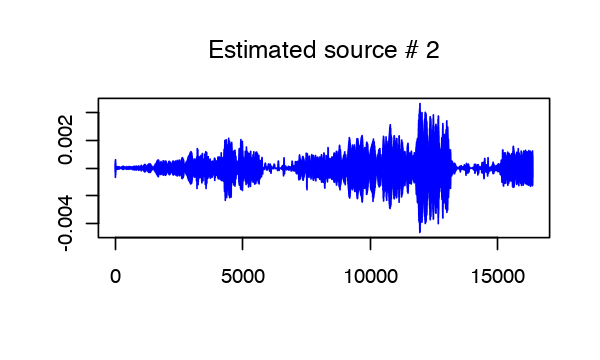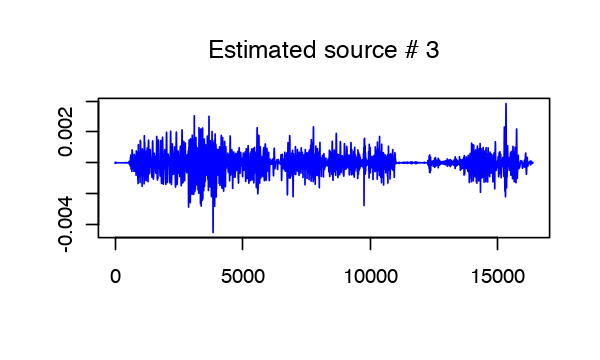One can listen to the recovered sources.

In :
i = 3
play(x[,i], rate=15000)

In :
play(xr[,i] * 1e4, rate=15000)

In [ ]:
<script>
$(document).ready(function(){$('div.prompt').hide();
});
</script>